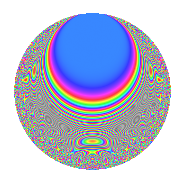# Properties

 Label 1502.2.aLevel 1502 Weight 2 Character orbit a Rep. character $$\chi_{1502}(1,\cdot)$$ Character field $$\Q$$ Dimension 63 Newforms 8 Sturm bound 376 Trace bound 3

# Related objects

## Defining parameters

 Level: $$N$$ = $$1502 = 2 \cdot 751$$ Weight: $$k$$ = $$2$$ Character orbit: $$[\chi]$$ = 1502.a (trivial) Character field: $$\Q$$ Newforms: $$8$$ Sturm bound: $$376$$ Trace bound: $$3$$ Distinguishing $$T_p$$: $$3$$

## Dimensions

The following table gives the dimensions of various subspaces of $$M_{2}(\Gamma_0(1502))$$.

Total New Old
Modular forms 190 63 127
Cusp forms 187 63 124
Eisenstein series 3 0 3

The following table gives the dimensions of the cuspidal new subspaces with specified eigenvalues for the Atkin-Lehner operators and the Fricke involution.

$$2$$$$751$$FrickeDim.
$$+$$$$+$$$$+$$$$13$$
$$+$$$$-$$$$-$$$$19$$
$$-$$$$+$$$$-$$$$18$$
$$-$$$$-$$$$+$$$$13$$
Plus space$$+$$$$26$$
Minus space$$-$$$$37$$

## Trace form

 $$63q - q^{2} + 2q^{3} + 63q^{4} - 2q^{5} - 2q^{6} + 4q^{7} - q^{8} + 67q^{9} + O(q^{10})$$ $$63q - q^{2} + 2q^{3} + 63q^{4} - 2q^{5} - 2q^{6} + 4q^{7} - q^{8} + 67q^{9} - 2q^{10} - 2q^{11} + 2q^{12} - 6q^{13} - 4q^{14} + 4q^{15} + 63q^{16} - 10q^{17} + 3q^{18} - 2q^{20} + 8q^{21} - 6q^{22} + 16q^{23} - 2q^{24} + 65q^{25} - 2q^{26} - 4q^{27} + 4q^{28} - 4q^{29} + 12q^{31} - q^{32} - 8q^{33} - 6q^{34} - 28q^{35} + 67q^{36} - 6q^{37} + 4q^{38} + 12q^{39} - 2q^{40} - 6q^{41} - 4q^{42} - 12q^{43} - 2q^{44} - 14q^{45} - 16q^{46} - 36q^{47} + 2q^{48} + 51q^{49} + q^{50} - 12q^{51} - 6q^{52} - 34q^{53} - 20q^{54} - 16q^{55} - 4q^{56} - 20q^{57} - 4q^{59} + 4q^{60} - 10q^{61} - 20q^{62} + 24q^{63} + 63q^{64} - 4q^{65} + 8q^{66} + 2q^{67} - 10q^{68} + 32q^{69} + 16q^{71} + 3q^{72} + 18q^{73} - 18q^{74} - 38q^{75} - 4q^{77} + 56q^{79} - 2q^{80} + 71q^{81} - 14q^{82} + 14q^{83} + 8q^{84} - 20q^{85} - 16q^{86} - 36q^{87} - 6q^{88} - 34q^{89} - 46q^{90} + 12q^{91} + 16q^{92} + 40q^{93} - 4q^{94} - 2q^{96} - 34q^{97} - 9q^{98} + 6q^{99} + O(q^{100})$$

## Decomposition of $$S_{2}^{\mathrm{new}}(\Gamma_0(1502))$$ into irreducible Hecke orbits

Label Dim. $$A$$ Field CM Traces A-L signs $q$-expansion
$$a_2$$ $$a_3$$ $$a_5$$ $$a_7$$ 2 751
1502.2.a.a $$1$$ $$11.994$$ $$\Q$$ None $$1$$ $$-2$$ $$2$$ $$4$$ $$-$$ $$+$$ $$q+q^{2}-2q^{3}+q^{4}+2q^{5}-2q^{6}+4q^{7}+\cdots$$
1502.2.a.b $$1$$ $$11.994$$ $$\Q$$ None $$1$$ $$1$$ $$2$$ $$4$$ $$-$$ $$+$$ $$q+q^{2}+q^{3}+q^{4}+2q^{5}+q^{6}+4q^{7}+\cdots$$
1502.2.a.c $$2$$ $$11.994$$ $$\Q(\sqrt{5})$$ None $$-2$$ $$0$$ $$-3$$ $$-3$$ $$+$$ $$+$$ $$q-q^{2}+(1-2\beta )q^{3}+q^{4}+(-2+\beta )q^{5}+\cdots$$
1502.2.a.d $$2$$ $$11.994$$ $$\Q(\sqrt{5})$$ None $$2$$ $$-1$$ $$2$$ $$-6$$ $$-$$ $$-$$ $$q+q^{2}-\beta q^{3}+q^{4}+q^{5}-\beta q^{6}+(-4+\cdots)q^{7}+\cdots$$
1502.2.a.e $$11$$ $$11.994$$ $$\mathbb{Q}[x]/(x^{11} - \cdots)$$ None $$-11$$ $$-4$$ $$1$$ $$-6$$ $$+$$ $$+$$ $$q-q^{2}+\beta _{10}q^{3}+q^{4}+(-1+\beta _{1}-\beta _{2}+\cdots)q^{5}+\cdots$$
1502.2.a.f $$11$$ $$11.994$$ $$\mathbb{Q}[x]/(x^{11} - \cdots)$$ None $$11$$ $$-11$$ $$-12$$ $$-9$$ $$-$$ $$-$$ $$q+q^{2}+(-1+\beta _{1})q^{3}+q^{4}+(-1-\beta _{7}+\cdots)q^{5}+\cdots$$
1502.2.a.g $$16$$ $$11.994$$ $$\mathbb{Q}[x]/(x^{16} - \cdots)$$ None $$16$$ $$13$$ $$4$$ $$7$$ $$-$$ $$+$$ $$q+q^{2}+(1-\beta _{1})q^{3}+q^{4}+\beta _{8}q^{5}+(1+\cdots)q^{6}+\cdots$$
1502.2.a.h $$19$$ $$11.994$$ $$\mathbb{Q}[x]/(x^{19} - \cdots)$$ None $$-19$$ $$6$$ $$2$$ $$13$$ $$+$$ $$-$$ $$q-q^{2}+\beta _{1}q^{3}+q^{4}-\beta _{3}q^{5}-\beta _{1}q^{6}+\cdots$$

## Decomposition of $$S_{2}^{\mathrm{old}}(\Gamma_0(1502))$$ into lower level spaces

$$S_{2}^{\mathrm{old}}(\Gamma_0(1502)) \cong$$ $$S_{2}^{\mathrm{new}}(\Gamma_0(751))$$$$^{\oplus 2}$$